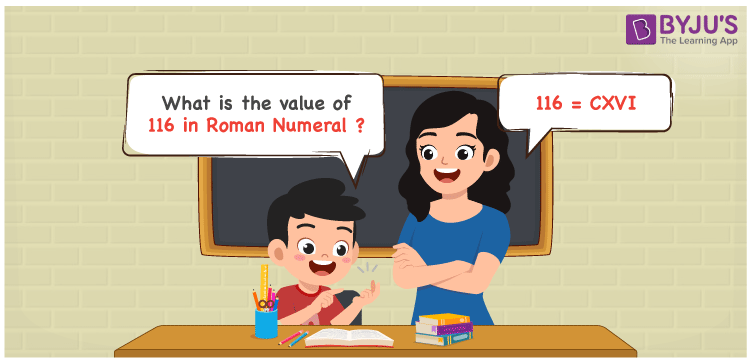# 116 in Roman Numerals

116 in Roman Numerals is CXVI. Any Roman numeral uses only the 7 primary symbols which are I, V, X, L, C, D, and M. Here C represents 100, X represents 10, V represents 5 and I represents 1. 116 can be obtained by adding the primary symbols C, X, V and I. For understanding the rules on Roman Numerals, you can refer to the link Rules to write Roman Numerals. The details on how to write 116 is given in the next section of this article.

 Number Roman Numeral 116 CXVI

## How to Write 116 in Roman Numerals?To convert 116 in Roman Numerals, we need to represent 116 as the sum of the fundamental symbols. 116 is nothing but 100 + 10 + 6, both 100 and 10 have the primary symbols but 6 does not. So 6 can be expressed as the sum of 5 and 1.

Representing it numerically;

116 = 100 + 10 + 6

116 = 100 + 10 + 5 + 1

116 = C + X + V + 1

116 = CXVI.

## Video Lesson on Roman Numerals## Frequently Asked Questions on 116 in Roman Numerals

Q1

### How to write the number 116 in Roman Numerals?

116 in Roman Numerals is written as CXVI.
Q2

### Do CXVI and IVXC represent Roman Numerals?

No. CXVI represents Roman Numerals and it is the number 116, where as IVXC is an invalid numeral.
Q3

### What is the year 116 in Roman numerals?

The year 116 in Roman Numerals is CXVI.